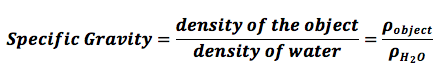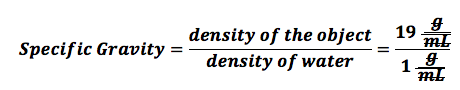Specific Gravity of the minerals - Global Epidemic Observational Studies Organization

# Specific Gravity of the minerals

Before going through the definition of specific gravity, we need to elaborate on the concept of density first. Density is the quantity of matter per unit volume of a substance. As the quantity of matter in different substances of equal volumes is different, therefore, the densities of different substances are quite different from one another. Every substance has a different quantity of mass in a specific volume. For example, 1 milliliter of water has a different mass from 1 milliliter of the volume of mercury. Similarly, masses per unite volume of solids also differ from one another. Some solids are much denser than others. In the case of studying densities, there is no benchmark. We don’t need to compare the density of one object with another’s to find the density of another object. The density of every object can be measured without depending on the measurement of the density of another object.But the case of specific gravity is quite different. Specific gravity is the ratio between the density of an object and a reference substance. The specific gravity can tell us, based on its value, if the object will sink or float in our reference substance. Usually, our reference substance is water which always has a density of 1 gram per milliliter or 1 gram per cubic centimeter. We need to compare the density of an object with that of water, meaning that how much the density of an object differs from the density of water. In the case of minerals, specific gravity is included in the most important physical properties of the minerals. It is a number, which represents the ratio of the weight of a mineral to the weight of equal volume of water. In other words, the difference of densities of mineral and water is known as the specific gravity of that mineral. Thus a mineral with specific gravity 4.0 is four times as heavy as water (water has a specific gravity=1). Similarly, a mineral with a specific gravity 5.5 means, that it is 5.5 times heavier than water. Or in other words, the object with specific gravity 5.5 has a density 5.5 times greater than the density of water. Specific Gravity explains, ”how much does an equal volume of a mineral is heavier or lighter than the same volume of water. A rough estimate of the specific gravity of minerals can be made by hefting them in our hands.

### The formula for Specific Gravity:

The formula for specific gravity, given that the reference substance is water, is the density of the object divided by the density of the water. Here, we use the Greek symbol Rho to indicate density.The specific gravity has no unit because the units of the numerator and the denominator are the same, so they just cancel each other out. Let’s look at an example. Here, the density of the object is 19 g/mL and the density of water is 1 g/mL. We cancel the unit g/mL because this unit is present in both the numerator and the denominator:### Determination of Specific Gravity:

The best method to determine the specific gravity of solid minerals is to measure the loss of weight of a body after immersing it in water. The weight lost by the object after immersing it into the water is equal to the weight of water of equal volume. The remaining weight of the immersed object is that which is higher than that of water. If W1 is the weight of the mineral, and W2 is the weight of the mineral after immersing it in the water. Same way, W2 will be equal to the weight of water of equal volume. Now we need to find the weight lost after immersing it in water, which can be found by W1-W2.

Specific Gravity= W1/(W1-W2)

Where W1 is the original weight of the object, W2 is the weight after immersion, while (W1-W2) is equal to the weight lost after immersion in water. k

### Walker’s Steel Yard:

It is a type of balance, which is used to determine the specific gravity of the objects. It is suitable in cases of large objects. This balance consists of a long graduated horizontal beam supported near one end on a knife edge, thus dividing the beam into a longer and a shorter arm. At the end of the longer arm, a vertical post is placed. It bears an index mark, which helps in aligning the beam in the horizontal position.

The mineral object, whose specific gravity is needed to be found out, is suspended by a very thin nylon thread from the shorter arm. It is moved along the graduated arm so as to bring the end of the arm opposite the fixed index mark and the position of the specimen on the arm is noted. It will be the actual weight of the mineral specimen. For example the first (actual) weight of the mineral speciment is 50 gm. Now bring a beaker filled with water underneath the object (mineral specimen) hanging with the arm of the balance. Raise the position of the beaker and allow the mineral to immerse in the water of the beaker. The weight of the mineral on the balance will surely reduce itself. Note the second reading of the weight. Assume that the weight (W2) after immersion is 40 gm. Now come to the formula;

Specific gravity = W1/ (W1-W2)

Specific gravity = 50/ (50-40)

Specific gravity = 50/10

Specific gravity = 5 (which means, that the object is 5 times heavier than water).

In case, if an object has a density equal to that of water. It will show its W2 equal to zero. Suppose we have a porous stone, which has an initial weight equal to 50 gm. After dipping it into the water, its wieght becomes zero, meaning that W2=0. By applying the formula;

Specific gravity = W1/(W1-W2)

Specific gravity = 50/(50-0)   (means that the object completely lost its weight on immersing in water).

Specific gravity = 50/50

Specific gravity = 1. (this is equal to the specific gravity of water)

### Jolley’s Spring Balance:The specific gravity of small fragments of a mineral is determined by Jolly’s spring balance. The instrument consists of a weak spring that is suspended vertically against a graduated mirror scale. The working mechanism of the Jolly’s spring balance is quite similar to that of Walker’s steelyard. In Jolly’s spring balance, first, we note the weight of the mineral specimen, which would be W1, and then note the second weight, W2, which we get after dipping the mineral specimen in the water. For example, W1 is 20 gm, W2 is 12 gm. The weight lost after immersion (W1-W2) will be 8 gm. Now putting the values in the formula;

Specific gravity = W1/ (W1-W2)

Specific gravity = 20/(20-12)

Specific gravity = 20/8

Specific gravity = 2.5

The specific gravity of the mineral specimen would 2.5, meaning that it is 2.5 times heavier than an equal volume of water.